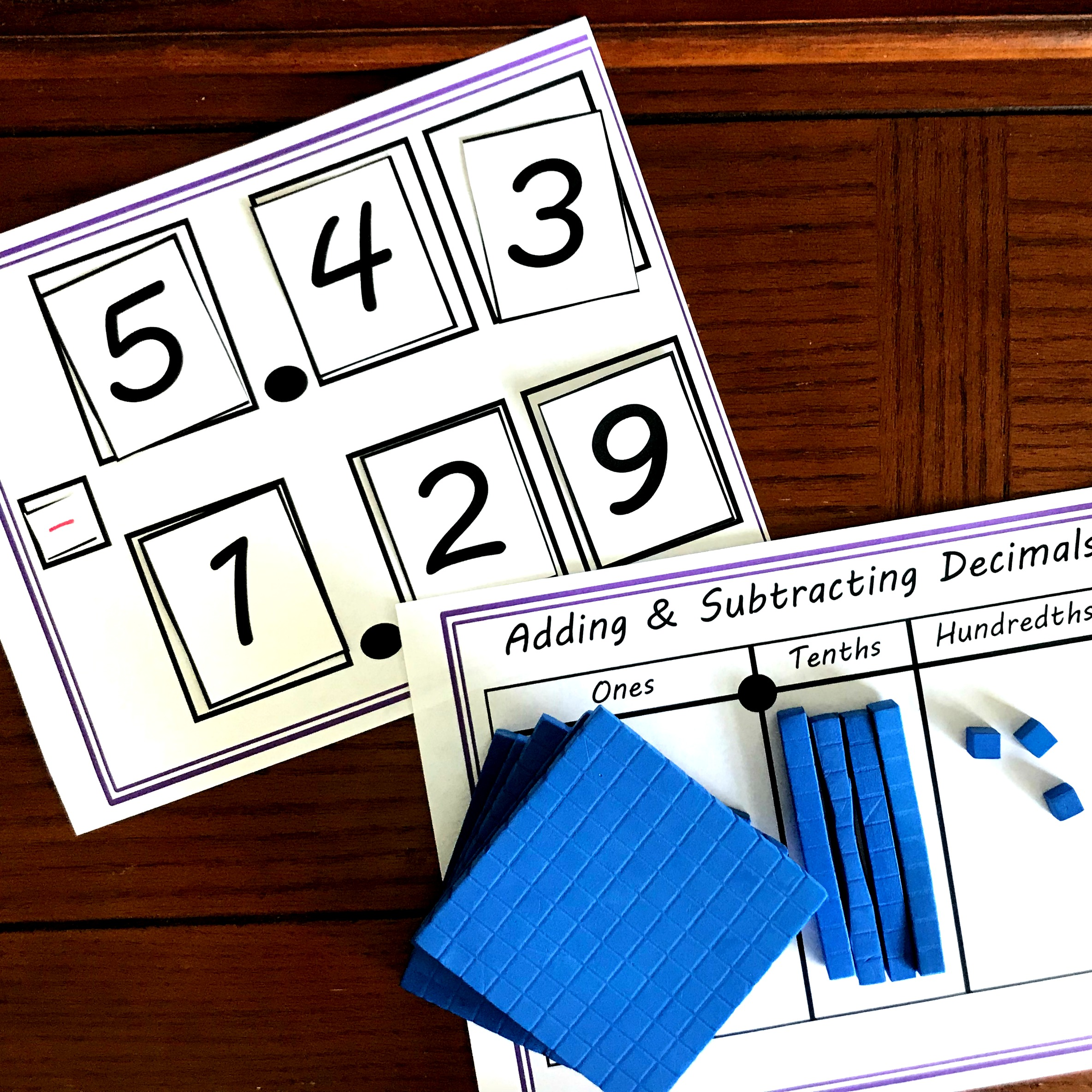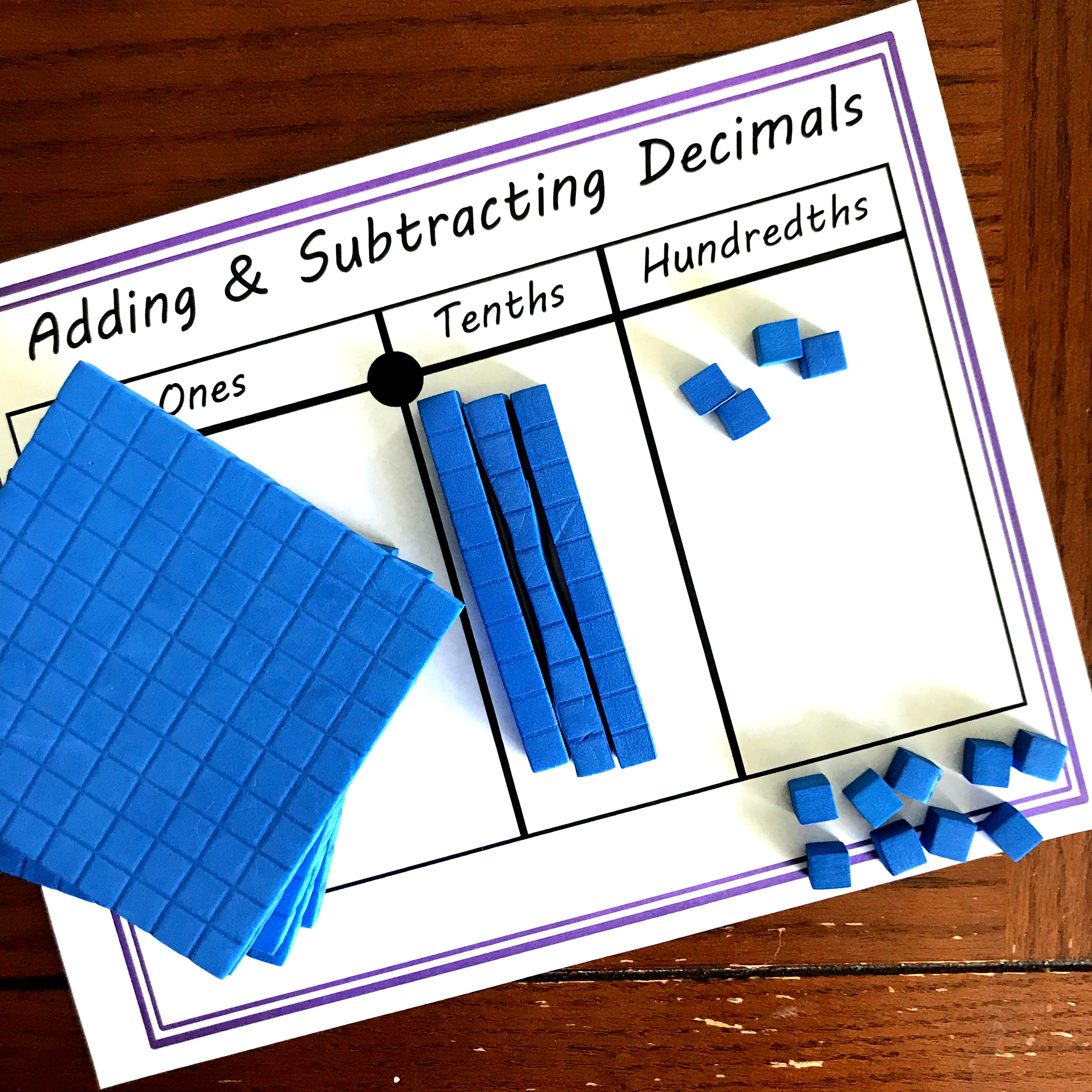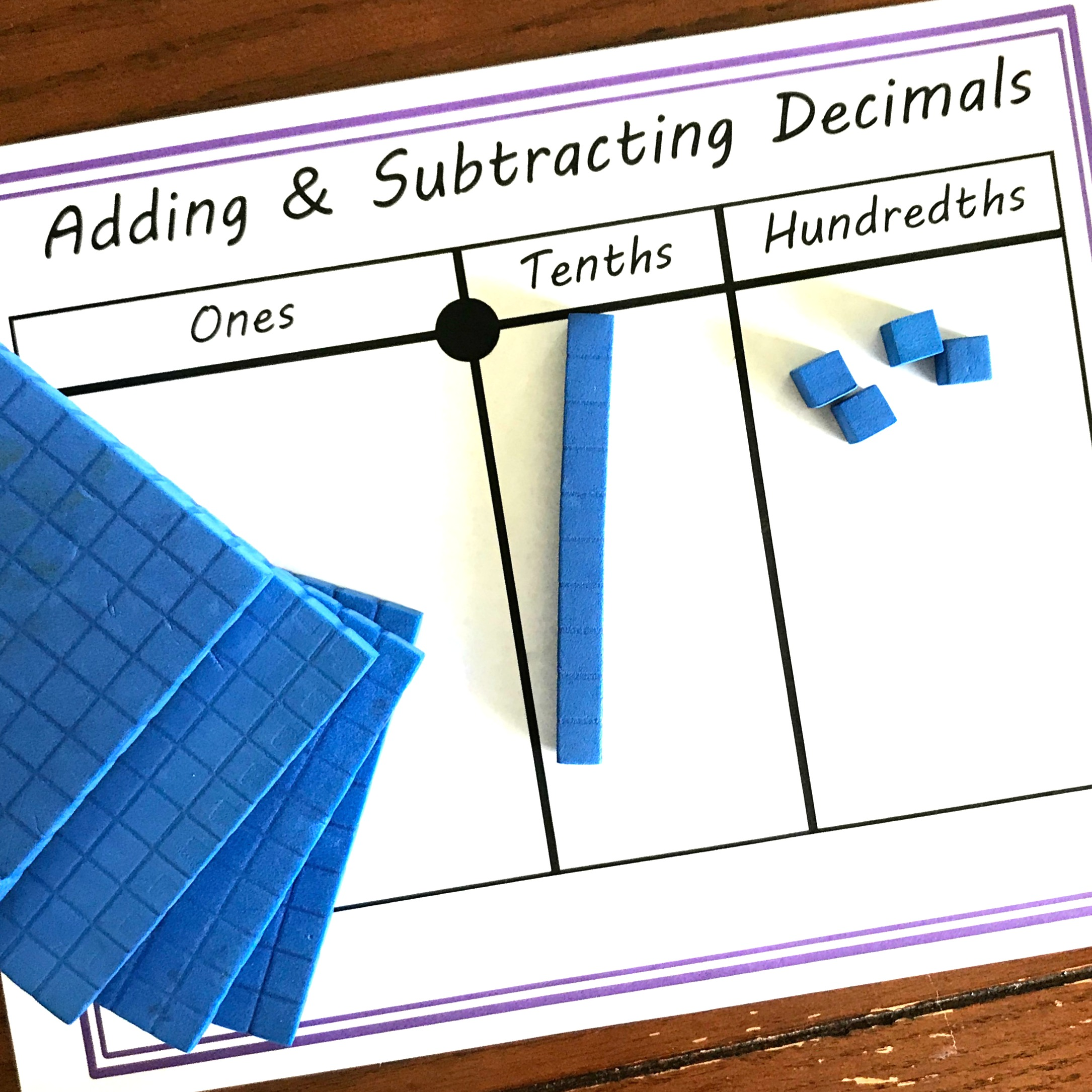Home » Math » Practice Addition and Subtraction Of Decimals With This Hands-On Activity

# Practice Addition and Subtraction Of Decimals With This Hands-On Activity

Using base ten blocks is a wonderful way to practice the addition and subtraction of decimals. This activity is low-prep and builds number sense.## Get your free printable by clicking on the large blue button at the end of the post!

Adding and subtracting with decimals can be hard for some kiddos. Children need to understand decimals and place value to be truly successful at this new concept.

So that is why I’m so excited to be teaming up with Math Geek Mama to dive deep into this subject. We will be exploring numerous ways to help children develop an understanding of decimals while adding and subtracting!

## Addition and Subtraction of Decimals

The first stop in our series is base ten blocks!

Base ten blocks are a wonderful way to teach adding and subtracting regular numbers. Once that knowledge is formed it is easy to take the same concept and move it into decimals…..just the value of the base ten blocks change.

This addition and subtraction of decimals activity only goes to the hundredths, so we will make the smallest base ten blocks, the cube, our hundredths. This means that the long, is our tenth. And the cube will represent our ones place.

## Prep-Work

This activity is simple to set up, and can be used over and over.

1. First, print off the mat, expression page and numbers.
2. Next, cut the numbers out.
3. Finally, gather up those base ten blocks!

Now it time to add and subtract.Teacher Created Resources Foam Base Ten Set (20617)To begin the activity, have your kiddo draw six numbers and place them on the expression mat. Then of course, we start by getting out our base ten blocks and placing them on the mat. In this example, we are adding 3.22 + 5.94, so we have to get out 3.21.

We have three ones, so we place three base ten blocks on the mat.

Then, we see that there are two tenths, so two longs go in the tenths place.

Finally, we add two hundredths, two cubes, into the hundredths place.

#### Step 2:

So this part is simple. We begin adding base ten blocks. We begin by adding four hundredths to the hundredths place, and voila we have five hundredths.

Now we move unto the tenths place. Looking at the problem we see that we need to add nine-tenths so now we have 11 tenths….and this is not Ok.

We need to do a little switching out, and this is very simple. All we have to do is take out 10 longs and place a flat in the ones place.#### Step 3:

All that is left is to add five ones or flats to the mats and then figure out how many blocks are in each place value.

We have nine flats, one long, and five cubes so my answer is 9.15!

## Subtracting Decimals:

Subtracting always seems to be a little harder, but when we introduce with base ten blocks….we build number sense that will help children be successful with these difficult concepts.

#### Step 1:Just like always we start by looking at the first number in the expression and getting that number out on the mat. So we take out five flats, four longs, and three tenths.

#### Step 2:Our next step is to subtract nine hundredths, but there are only three hundredths, so some regrouping is needed. We simply do this by taking a long off the mat and then replacing that long with 10 cubes. And we add those 10 cubes to the hundredths. NOW, we can subtract nine hundredths, and we end up with four hundredths.#### Step 3:Our next step is to take away two tenths. This time it is easy because we have three tenths, so three minus two-tenths is one tenth.

#### Step 4:

Finally, we move onto our ones. All we need to do here is take away one flat from the one’s place!#### Step 5:

After we have subtracted from each place value, there is only one step left….see what is left.And, as you can see there are four ones, one-tenth, and four hundredths. So my answer is 4.14!!

Practicing addition and subtraction of decimals is essential, and when we add base ten blocks it helps children to visualize and understand decimals better!!!

You’ve Got This

Rachel

This is a subscriber freebie. If you are already a subscriber, check the most recent email for the latest password. If you aren’t a subscriber, sign up below and the password will be provided in the first email you receive. You will also get \$2 off to use the You’ve Got This Store.

You’ll find it under the decimal section!